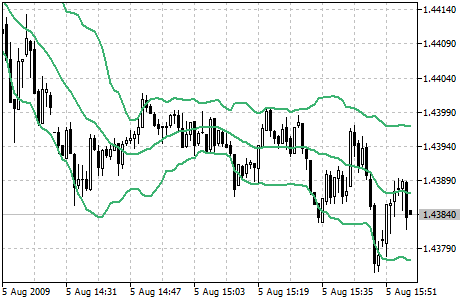# 布林带®

1. 由于波动减小带宽缩小易于发生价格突变；
2. 如果价格在上面的带间断，当前的趋势会继续；
3. 如果带内的凹凸追随带外的，会出现反方向趋势；
4. 从一条带线开始的价格移动通常会到达相反方向。## 计算

ML = SUM (CLOSE, N) / N = SMA (CLOSE, N)

TL = ML + (D * StdDev)

BL = ML - (D * StdDev)

SUM (..., N) N周期求和;
CLOSE 收盘价;
N 计算中使用到的周期;
SMA is a 简单移动平均;
SQRT 平方根;
StdDev - 标准差。

StdDev = SQRT (SUM ((CLOSE ― SMA (CLOSE, N))^2, N)/N)